tracker890 Source h
No Effort - Member warned that some effort must be shown
Dear Everyone：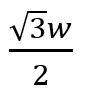?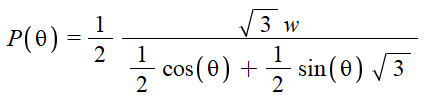Thank you for your time and consideration.

Staff Emeritus
Gold Member
You're trying to prove that ##\sqrt{3} w/2## is the smallest value P can take? What have you done so far to try to solve this? We can help identify what the next step is easier if we see what your work is so far.

•mfb
tracker890 Source h
Dear Office_Shredder
Thank you for reminding, and this problem has been solved.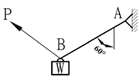Problem：
Determine the Pmin for the sys equilibrium.

sol/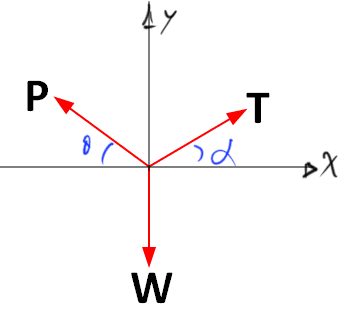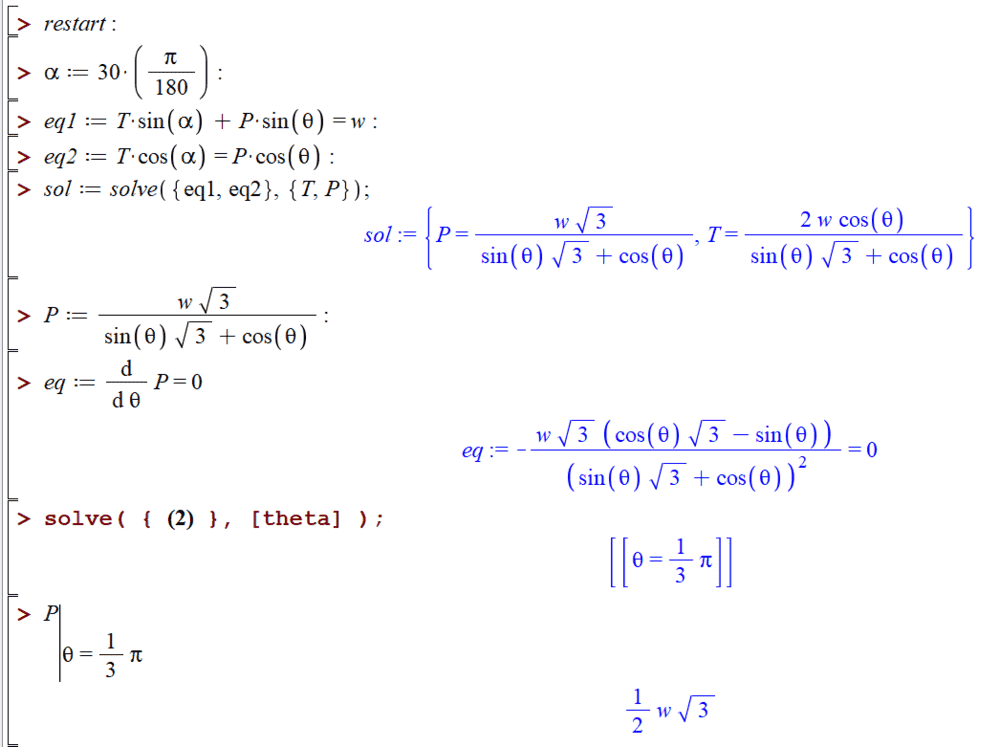#### Attachments

Last edited:
Master1022
Dear Everyone：

Thank you for your time and consideration.

I know this has been solved now, but another method to do this (other than differentiation) is to notice that the denominator can be expressed as a double-angle formula. The coefficients $\sqrt 3 /2$ and $1/2$ seem to stand out to me. For example:

$$\frac{1}{2} cos\theta + \frac{\sqrt 3}{2} sin \theta = sin(\theta + \frac{\pi}{6})$$
We can now see that we want the minimum of $P$ which occurs when the denominator is a maximum. Max(sin) = 1 and thus we get the answer as required (and we can see that $\theta = \pi / 3$ for the sin() to equal 1)

•tracker890 Source h, etotheipi and PeroK
Mentor
An even shorter option: If two sine waves are shifted by pi/2 (just like sine and cosine are) then they can be combined to a single sine function by adding the individual amplitudes in quadrature. As formula: ##a\sin(\theta)+b\cos(\theta) = \sqrt{a^2+b^2} \sin(\theta+x)## with some phase x we don't need to care about. This function has a maximum of ##\sqrt{a^2+b^2}##. That means we just need to calculate ##\sqrt{\left(\frac{1}{2}\right)^2+\left(\frac{\sqrt{3}}{2}\right)^2} = \sqrt{\frac 1 4 + \frac 3 4}=1##.

•tracker890 Source h